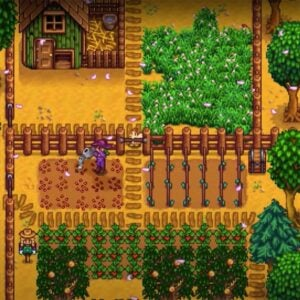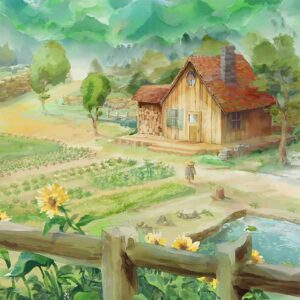# Eric Barone

2 Music Sheets

Eric Barone

• ###### Genres:

Game developer and composer.

Credit: Wikipedia

## Artist's Music Sheets

•[a8]|s|[wd]|f|[a8] s d f [wa]|p|[a8]|s|[wd]|f|[a8]sas d f [wa]|p|[a6]|s|[hd0]|f|[da6] s d f [fa0]|p|[wtoe6] | [a8]|s|[wd]|f|[a8] s d [kf] [wha]|p d [fa8]|s|[wd]|f|[a8]sas d [kf] [wha]|p d [sp6]|a|[d0]|f|[a6] s d h [z0] j h k [l4] k h d [s8] a o u [tq4] | [t8]|a|i|u|p|a|i|[us] y [ta8]|a|i|u|p|a|i|[up] y [tsqe6]|a|i|u|p|a|i|[uo] y [tqea]|a|i|u|p|a|d | [ta8]|a|[oi]|u|[to]|a s [od] f a k [sh8]|a|i|u|r|a s [od] f h k [lh6] k h d [us] a o u [tp]|a|u|y|[tq4] | t | [tq4]|p|a|s|[a8]|s|[wd]|f|[a8] s d [kf] [wha]|p d [fa8]|s|[wd]|f|[a8]sas d [kf] [wha]|p d [sp6]|a|[d0]|f|[a6] s d h [z0] j h k [l4] k h d [s8] a o u [tq4] | [uto8] | z|l | [uoa0] | h|G | [sqpi] | c|x | j|[uto8] | [uto8] | 5|[shf8]|f|d|f|h | x|m|[khf0]|h|G|h|k | b|n|[qljg]|j|h|g|h | s | [d8] f r t y|u|o|p|opo|[y5]|[uto81]|u|y|u|[uto] | [xl]|l|[uoa30]|o|I|o|[uoa] | [jf]|k|[sqpi4]|p|o|i|[uo] | t | [t81]|r t y|u|o|p|opo|[y5]|[t81]

Level: 6
Length: 02:45
Intermediate

#### Eric Barone

•7 ( Q Qr r7 0 W Wr r7 ( Q Qr r[YD7]| |7 ( Q Qr r7 0 W Wr r7 ( Q Qr r7| |[D7] ( Q Q[rG]| [f7] 0 [WD] W[rf]| [G7] ( Q Q[rD]| [a7] ( Q Qr| [D7] ( Q Q[rG]| [f7] 0 [WH] W[rG]| [S\$] ^ * *Q| \$ ^ * Q E Q [D7] ( Q Q[rG]| [f7] 0 [WD] W[rf]| [G7] ( Q Q[rD]| [a7] ( Q Qr S [D7] ( [SQ] Q[ra]| [O7] 0 W W[rI]| [a7] ( Q Qr| 7 ( Q r Y I [I7]aD( Q Q[rG]| [f7]Gf0 [WD] W[rf]| [G7] ( Q Q[rD]| [a7] ( Q Qr| [I7]aD( Q Q[rG]| [f7] 0 [WH] W[rG]| [I\$]PS^ * *Q| \$ ^ * Q E Q [I7]aD( Q Q[rG]| [f7]Gf0 [WD] W[rf]| [G7] ( Q Q[ra]| [H7] 0 [WG] W[rf]| [D7] ( [SQ] Q[ra]| [O7] 0 W W[rI]| [a7] ( Q ( r Q Y r I Y a P [rk] Y I a I [YJ] [rH] u O a O [uf] [rG] Y I a I [YD] [SQ] E T I [aT] [SE] [rD] Y I a [OI] Y [rf] u [OD] a [fO] u [rG] Y I a IG[YJ]k[QC] E T I T E [rk] Y I a I [YJ] [rH] u O a [fO] u [rG] Y I a I [YH] [QJ] E T I T [GE] [k(] W r Y r [WL] [J(] Q E T [GE] Q [rk] Y I a I Y r Y I a I Y [rG7]DSaGDSaGDSa[D7]SaIDSaIDSaI[aW%]POYaPOYaPOY[O30]IurOIurOIur[rQ7]rY

Level: 5
Length: 01:54
Intermediate